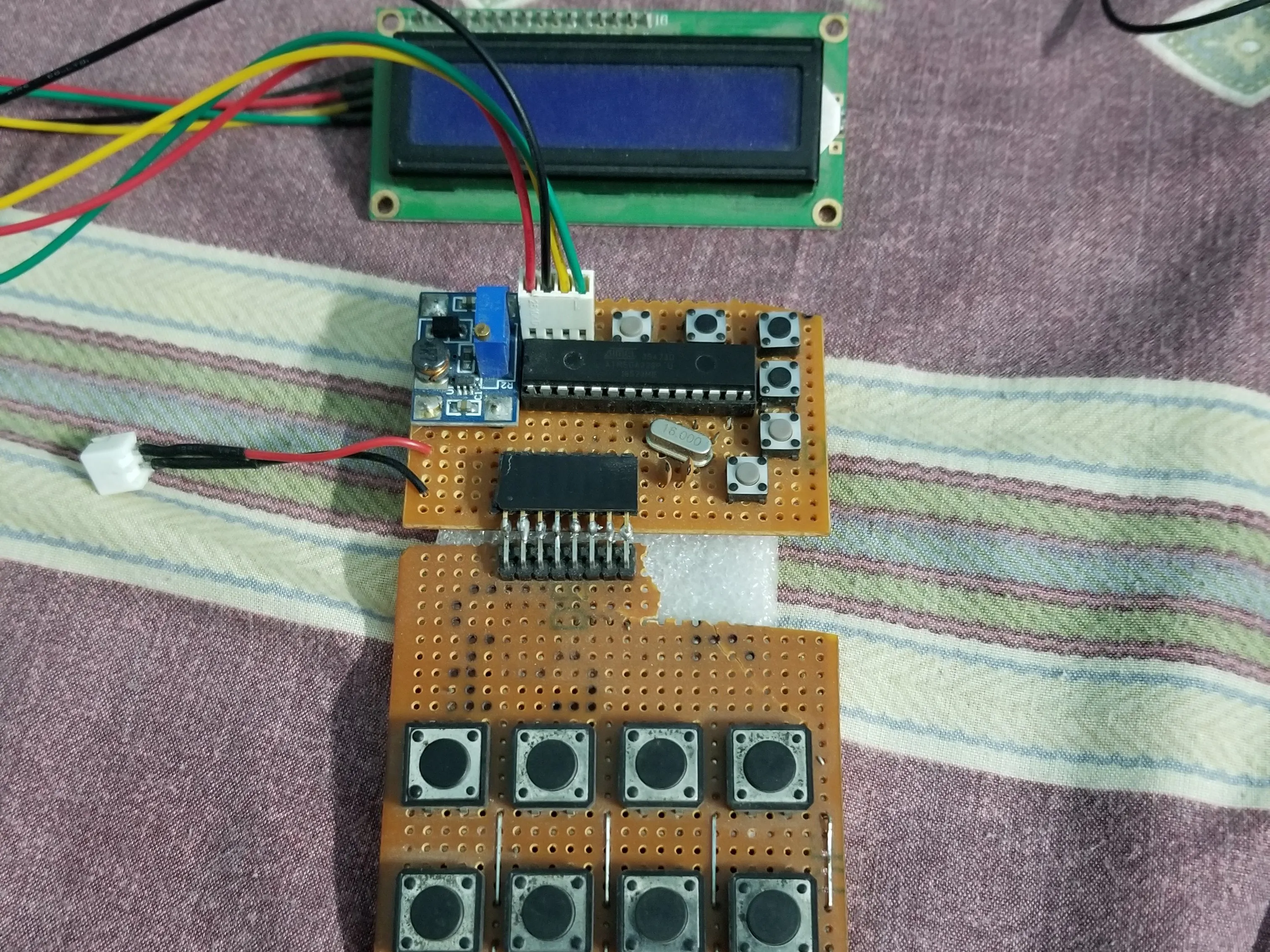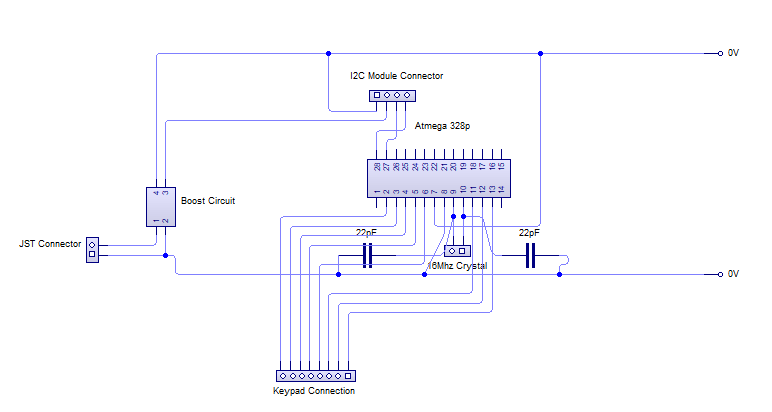Project tutorial# Arduino Based Digital Calculator

This project is based on digital calculator for converting whole number (decimal) to Binary, Hexa, and Octa decimal system

• 12 views
• 1 comment
• 0 respects

## Apps and online services

I needed a small calculator to convert any whole number into another number system without any problem.

For this reason, I chose to create this project. This project consists of DIY based keypad that works perfectly, an Arduino programmed 328p controller, LCD with I2C module, 3.3v to 5v boost circuit and perf-boards.

Let's start with the keypad, I have used 8 push buttons which are arranged in such a way that they look like a real Arduino keypad, I have used some stapler pins that act as a jumper and also it acts as a conductor.

These pins are connected to the Atmega 328p microcontroller with the help of L-shaped male headers. As you can see in the above photos that four pins act as rows pins while the other four pins act as columns.

Now, let's discuss the mainboard. The mainboard consists of a micro-controller, a 16Mhz crystal oscillator, few buttons (they are dummy, but they can be configured as your requirement), two 22pF non-polar capacitors, an I2C connector for LCD and a boost circuit as shown in the circuit below.

As for the LCD, the I2C module is directly soldered on the LCD PCB, and there is no gap between them. The mainboard is powered by the 3.3v LiPo battery, the boost circuit converts the low voltage to the high voltage since there is the potentiometer connected on the boost circuit which varies the voltage at the output. So by varying it, the voltage can be changed, this micro-controller only requires about 5V, so the voltage is varied to 5V on the output. This voltage is also supplied on the I2C module this turns on the LCD.

The I2C module on the LCD consists of four pins which are connected in the following ways:5V -------- 5V (output of boost circuit)

GND ---- GND (output of the boost circuit)

SCL ------ A5 (SCL of Atmega 328p) Arduino

SDA ----- A4 (SDA of Atmega 328p) Arduino

The programming of this project is simple and easy.

``#include <LiquidCrystal_I2C.h>#include <Keypad.h>``

Above these two libraries are included for this project.

The coding attached, please see it and you will understand it easily.

## Code

##### Digital CalculatorC/C++
```#include <LiquidCrystal_I2C.h>

LiquidCrystal_I2C lcd (0x27, 16, 2);

const byte rows = 4;
const byte cols = 4;

char hexKeys [rows][cols] = {

{'1', '2', '3', 'A'},
{'4', '5', '6', 'B'},
{'7', '8', '9', 'C'},
{'*', '0', '#', 'D'}
};

byte rowPins[rows] = {4, 5, 6, 7};
byte colPins[cols] = {0, 1, 2, 3};

long number = 0;

char dynamicKeys;
String string = "";

boolean enterkey_state;
boolean binButton_state;
boolean hexButton_state;
boolean octButton_state;
boolean decButton_state;

void setup()
{
lcd.begin();
lcd.backlight();

lcd.clear();
lcd.setCursor(0, 0);
lcd.print("Initializing");
for (int i = 12; i <= 16; i++)
{
delay(200);
lcd.print("*");
}
delay(3000);
lcd.clear();

lcd.setCursor(0, 0);
lcd.print("Digital");
lcd.setCursor(0, 1);
lcd.print("Calculator");

delay(3000);
lcd.clear();
}

void loop()
{
enterkey_state = false;
binButton_state = false;
hexButton_state = false;
octButton_state = false;
decButton_state = false;

dynamicKeys = kpd.getKey();

if (dynamicKeys)
{
lcd.clear();
lcd.setCursor(0, 0);
lcd.print("|>");
string = string + dynamicKeys;
lcd.print(string);
number = string.toInt();
}

if (dynamicKeys == 'A')
{
octButton_state = true;

lcd.clear();
lcd.setCursor(0, 0);
lcd.setCursor(0, 1);
lcd.print("|>");
lcd.print(number, OCT);
}
else if (dynamicKeys == 'B')
{
binButton_state = true;

lcd.clear();
lcd.setCursor(0, 0);
lcd.print("Binary");
lcd.setCursor(0, 1);
lcd.print("|>");
lcd.print(number, BIN);
}
else if (dynamicKeys == 'C')
{
hexButton_state = true;

lcd.clear();
lcd.setCursor(0, 0);
lcd.setCursor(0, 1);
lcd.print("|>");
lcd.print(number, HEX);
}
else if (dynamicKeys == 'D')
{
decButton_state = true;

lcd.clear();
lcd.setCursor(0, 0);
lcd.print("Decimal");
lcd.setCursor(0, 1);
lcd.print("|>");
lcd.print(number, DEC);
}
else if (dynamicKeys == '#')
{
enterkey_state = true;

lcd.clear();
lcd.print("Entered Number");
lcd.setCursor(0, 1);
lcd.print("|>");
lcd.print(number);
}
else if (dynamicKeys == '*')
{
number = 0;
string = "";
lcd.clear();
lcd.setCursor(0, 0);
lcd.print("Enter Number");
lcd.setCursor(0, 1);
lcd.print("|>");
}
}
```

## Schematics#### Published on

April 15, 2020
See similar projects
you might like

#### Nipkow Disk Based Digital Display Device

Project showcase by christopheArduino

• 17,545 views
• 57 respects

#### Digital Clock

Project in progress by Team SharKode

• 8,928 views
• 31 respects

#### Tri-Mode Digital Clock with ATtiny85 and RTC

Project tutorial by LAGSILVA

• 9,685 views
• 26 respects

Project tutorial by Team evive: an opensource embedded platoform

• 7,653 views
• 18 respects

#### Digital Oscilloscope Experiment Based on Arduino

Project tutorial by Andrew85

• 7,532 views